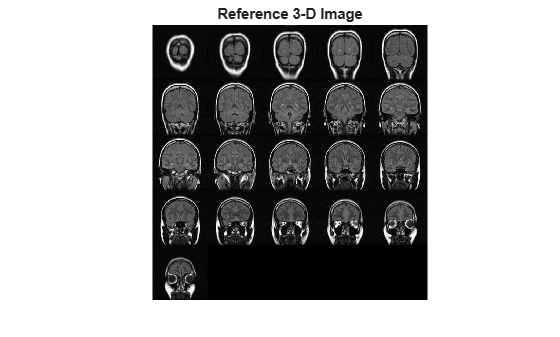# imhistmatchn

Adjust histogram of N-D image to match histogram of reference image

## Syntax

``B = imhistmatchn(A,ref)``
``B = imhistmatchn(A,ref,nbins)``
``````[B,hgram] = imhistmatchn(___)``````

## Description

example

````B = imhistmatchn(A,ref)` transforms the N-D grayscale image `A` and returns output image `B` whose histogram approximately matches the histogram of the reference image `ref`. Both `A` and `ref` must be grayscale images, but they do not need to have the same data type, size, or number of dimensions.```
````B = imhistmatchn(A,ref,nbins)` uses `nbins` equally spaced bins within the appropriate range for the given image data type. The returned image `B` has no more than `nbins` discrete levels.If the data type of the image is:`single` or `double`, the histogram range is [0, 1].`uint8`, the histogram range is [0, 255].`uint16`, the histogram range is [0, 65535].`int16`, the histogram range is [-32768, 32767].```
``````[B,hgram] = imhistmatchn(___)``` returns the histogram of the reference image `ref` used for matching in `hgram`. `hgram` is a 1-by-`nbins` vector, where `nbins` is the number of histogram bins.```

## Examples

collapse all

Load an N-D grayscale image into the workspace. Also load a grayscale image to provide a reference histogram.

```load mri D load mristack```

Display the original volume as slices.

```figure montage(D,'DisplayRange',[]) title('Original 3-D Image')```Reshape the reference as a stack of grayscale slices for display.

`ref = reshape(mristack,[256,256,1,21]);`

Display the reference volume as slices. To display correctly on the screen, the reference volume is downsized by a factor of 0.5 using `imresize`.

```ref_downsized = imresize(ref,0.5); figure montage(ref_downsized,'DisplayRange',[]) title('Reference 3-D Image')```Match the histogram of `D` to the histogram of the fullsize `ref`.

`Dmatched = imhistmatchn(D,ref);`

Display the output. Observe that the brightness levels of the output more closely match the reference image than the original image.

```figure montage(Dmatched,'DisplayRange',[]) title('Histogram Matched MRI')```## Input Arguments

collapse all

Input image to be transformed, specified as an N-D grayscale image.

Data Types: `single` | `double` | `int16` | `uint8` | `uint16`

Reference image whose histogram is the reference histogram, specified as a grayscale image. The reference image provides the equally spaced `nbins` bin reference histogram which output image `B` is trying to match.

Data Types: `single` | `double` | `int16` | `uint8` | `uint16`

Number of equally spaced bins in reference histogram, specified as a positive integer. `nbins` also represents the upper limit of the number of discrete data levels present in output image `B`.

Data Types: `double`

### Output Arguments

collapse all

Output image, returned as an N-D grayscale image. The output image is derived from image `A` whose histogram is an approximate match to the histogram of input image `ref` built with `nbins` equally spaced bins. Image `B` is of the same size and data type as input image `A`. Input argument `nbins` represents the upper limit of the number of discrete levels contained in image `B`.

Data Types: `single` | `double` | `int16` | `uint8` | `uint16`

Histogram counts derived from reference image `ref`, returned as a 1-by-`nbins` vector.

Data Types: `double`